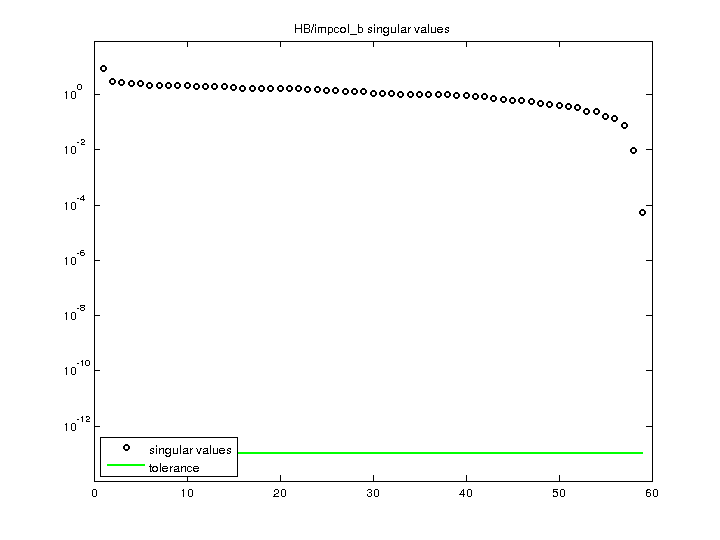Matrix: HB/impcol_b

Description: UNSYMMETRIC MATRIX - CAVETT'S PROCESS (CHEM ENG),1982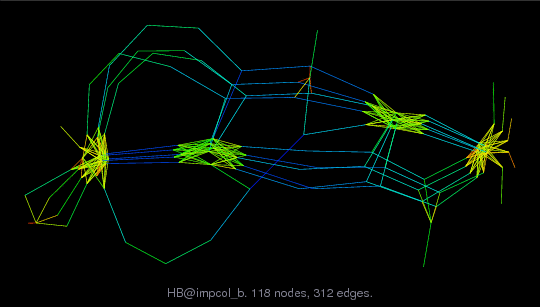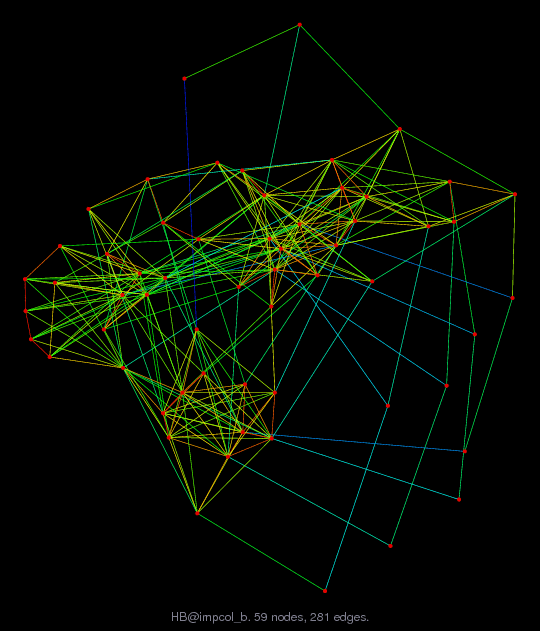(bipartite graph drawing) (graph drawing of A+A')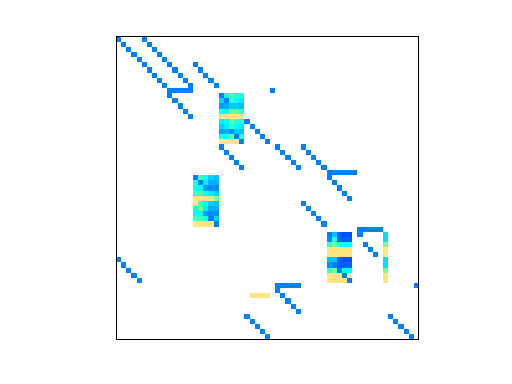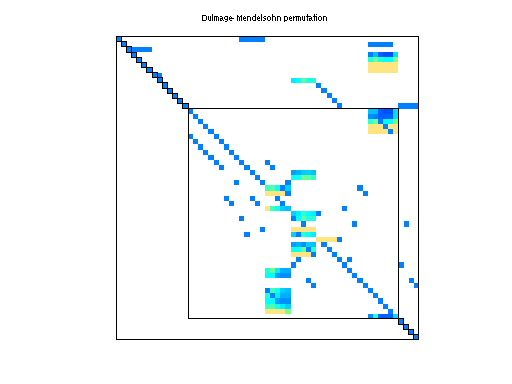• Matrix group: HB
• download as a MATLAB mat-file, file size: 2 KB. Use UFget(172) or UFget('HB/impcol_b') in MATLAB.

 Matrix properties number of rows 59 number of columns 59 nonzeros 271 structural full rank? yes structural rank 59 # of blocks from dmperm 19 # strongly connected comp. 1 explicit zero entries 41 nonzero pattern symmetry 9% numeric value symmetry 0% type real structure unsymmetric Cholesky candidate? no positive definite? no

 author D. Bogle editor I. Duff, R. Grimes, J. Lewis date 1982 kind chemical process simulation problem 2D/3D problem? no

 Ordering statistics: result nnz(chol(P*(A+A'+s*I)*P')) with AMD 561 Cholesky flop count 7.2e+03 nnz(L+U), no partial pivoting, with AMD 1,063 nnz(V) for QR, upper bound nnz(L) for LU, with COLAMD 235 nnz(R) for QR, upper bound nnz(U) for LU, with COLAMD 468

Note that all matrix statistics (except nonzero pattern symmetry) exclude the 41 explicit zero entries.

 SVD-based statistics: norm(A) 8.6395 min(svd(A)) 5.28815e-05 cond(A) 163375 rank(A) 59 sprank(A)-rank(A) 0 null space dimension 0 full numerical rank? yes

 singular values (MAT file): click here SVD method used: s = svd (full (A)) ; status: ok